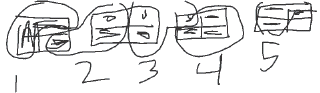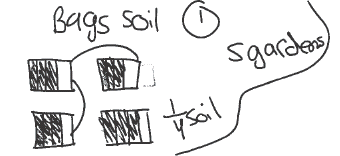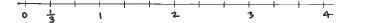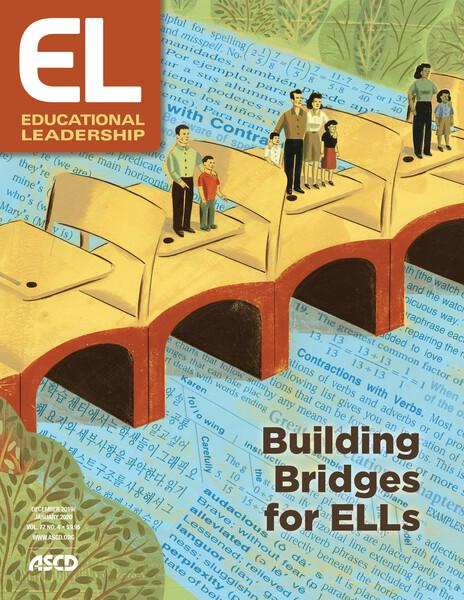Making Mathematical Thinking Visible - ASCDDecember 1, 2019

# Making Mathematical Thinking Visible

For English language learners, diagrams can be a powerful tool to develop and communicate mathematical understanding.Instructional StrategiesImagine being a 6th grade student who is still learning English, sitting in a mathematics classroom and trying to navigate the lesson. You might wonder: What is the teacher saying I should do? Did my classmates solve it the way I did? Will the other students laugh at me when I try to explain how I solved the problem?

Now imagine being a teacher in that same classroom. You might be asking yourself: What can I say so that all my students will understand what to work on? How do I know that all my students understand how to solve this problem? How can I help students share their mathematical thinking in productive ways?

These challenging questions are part of the hard work of mathematics instruction, and they are important to consider for all students. But they are especially pertinent to working with students who are learning English. Unfortunately, students who are English language learners 1 too often become invisible in math classrooms because they lack proficiency in English and don't have opportunities to communicate their mathematical thinking. It doesn't have to be this way.

For the past decade, we have studied the role that mathematical diagrams can play in giving ELLs more productive mathematics experiences. We have collaborated with hundreds of middle-grades teachers across the country to investigate how visual representations such as area models, number lines, and geometric figures are used by students and how they can facilitate ELLs' mathematical reasoning and communication. By making mathematical thinking visible, we've found, diagrams can play a central role in enriching mathematics education for ELLs.

## Illustrating the Problem

Research has documented that mathematical diagrams can be helpful for all students and for ELLs in particular. Mathematics instruction that uses multiple types of mathematical representations reinforces the learning of concepts, processes, language, and norms of mathematical communication (Chval & Chávez, 2011; Woodward et al., 2012). Diagrams are of particular value because they can illustrate a task's mathematical structure and help connect the text of a word problem to abstract concepts or the symbolic notation for solving it (Ng & Lee, 2009). Diagrams are also useful tools for supporting mathematical reasoning, problem solving, and communication. Furthermore, diagrams are a way that teachers and classmates can see and hear what ELLs are thinking and doing in their math work, thus positioning ELLs as active participants in mathematics lessons (Driscoll, Nikula, & Neumayer DePiper, 2016).

To illustrate how diagrams can facilitate and make visible ELLs' quantitative reasoning and mathematical communication, we offer three examples drawn from work by students we've observed.

Jan, a 6th grade ELL, worked on the following task: "Maureen bought 4 bags of soil for her gardens. Each garden needs 3/4 of a bag of soil. How many flower gardens can she fill?" 2 She drew a diagram (Figure 1) 3 , and then explained it: "First, I drew the 4 bags then I cut them into fourths. I shaded in three for each one and circled it. That's one garden. That's two gardens, that's three gardens, that's four gardens, and that's five gardens. So, she can fill completely five gardens and she will have 1/4 of a bag left over."

#### Figure 1. Jan's Diagram of the Flower Garden TaskNote that mathematical relationships are prominent in Jan's diagram and explanation. Her work presented a repeated subtraction approach to division, subtracting 3/4 repeatedly from the four bags, and counting the total number of times she can count 3/4 from the four whole bags. The diagram helped her to keep track of the quantities and relationships between quantities. In this sense, we can say that the practice of diagraming supported Jan in becoming more proficient in the mathematical practice of reasoning quantitatively and abstractly—a key mathematical practice under the Common Core standards (NGA & CCSSO, 2010).

Sam, another 6th grade EL, took a different approach with his diagram on the same problem:

#### Figure 2. Sam's Diagram of the Flower Garden TaskHe explained: "I made four flower gardens, and I divided by fourths. Then I shaded in 3/4 of a garden because […] the four boxes represent the fourths and the part that's shaded in represents the 3/4." When the teacher asked where the fifth garden came from, Sam responded: "When I shaded in with 3/4 in each group, I found there was 1 left over in each group because I used all the 3/4 I could find. I took the 3/4 from the three other groups and made them into one group and I know there was one left over."

While Sam mistakenly said "I made four flower gardens," he correctly labeled his four rectangles as "bags of soil," and was able to use the diagram to link quantities in the task to mathematical operations needed for a solution by attending to the mathematical structure of the problem, another key mathematical practice under the Common Core standards (NGA & CCSSO, 2010).

In another classroom, two 6th grade ELLs, Carmine and Luis, worked on a word problem for which they needed to figure out what 4 ÷ 1/3 equals. The students used a number line (Figure 3), and as they proceeded, Carmine pointed at the line, using her finger to show jumps for each 1/3, starting at 0 and moving her finger closer to 4 with each jump, while Luis counted in Spanish, "Uno, dos, tres…."

#### Figure 3. Number line for the task, 4 ÷ 1/3Carmine and Luis also had a set of printed cards with sentence starters such as "This diagram shows …" and "I like this diagram because …" to help them structure explanations during their pairs work. Using these sentence starter cards, the students explained, "This diagram shows 12 jumps," and "I like this diagram because I see all the one-thirds."

In this case, the diagram, in combination with their use of their first language to support thinking through a problem and sentence starters to frame their mathematical explanations, facilitated the students' conceptualization of the task and provided the teacher a clear representation of their thinking.

## Supporting Sensemaking in Mathematics

For Jan, Sam, Carmine, and Luis, diagrams served as a thinking tool, helping them to navigate the quantities given in their word problems and find mathematical relationships to help solve the problem. Diagrams can be instrumental in facilitating this kind of sensemaking and the selection and modification of a solution strategy (Ng & Lee, 2009).

Of course, we also want students to acquire and use the various forms of algebraic representations, but it is possible to build toward such representations using diagrams. One of the research-based recommendations for math instruction is to teach students how to use diagrams, which can make them more effective problem solvers, rather than just focusing on algebraic representations (Woodward et al., 2012). By focusing on diagrams before, or in addition to, algebraic representations, students have more opportunities to engage in mathematical thinking.

Diagrams represent the critical mathematical content—for example, fraction division in the examples above—and they call students' attention to the quantities given in a problem, as well as the relationships between quantities. Diagrams can also provide a structure for understanding a term, phrase, concept, or problem context because they can link the words that set out a problem with the calculations that move toward a solution. In this sense, they can provide an important bridge when students struggle with the words-to-symbols-to-numbers transitions.

As a tip for teachers, we have also found in our work that diagrams don't have to be created by the students to serve as powerful referents for mathematical thinking. Intentionally chosen or designed diagrams, or fictitious student work that presents one way of solving a task without suggesting it is the only method, can also encourage student reasoning and expression (Booth et al., 2013).

## Supporting Student Communication

In addition to supporting students' sensemaking, diagrams can facilitate mathematical communication and language development. To meet ELLs' needs, math teachers must use practices that "specifically address the language demands of students who are developing skill in listening, speaking, reading, and writing in a second language while learning mathematics" (Celedón-Pattichis & Ramirez, 2012, p. 1). We have found that integrating mathematical diagrams into instruction—by having students both create them and analyze ones that others have created—provides students artifacts they can use to talk about the mathematical relationships they see, describe their problem-solving processes, and deepen their understanding of embedded mathematical concepts.

Through our work with ELLs, we've seen how students' diagrams help communicate the richness of their mathematical thinking. Diagrams help them showcase their mathematical thinking to the teacher and other students, without having to rely only on words or having to know the "correct" words. In this manner, diagrams promote student agency and mathematical authority. For Jan, Sam, Carmine, and Luis, all of whom have low-intermediate or beginner English-proficiency levels, their diagrams were resources for communicating their thinking in addition to serving as tools to support thinking.

As shown in the example of Carmine and Luis above, ELLs can benefit from specific language supports integrated with diagram use. Sentence starters, in particular, can help elicit student explanations about their diagrams. In providing structures for students' writing and speaking, sentence starters can focus students' attention on specific mathematical relationships in a diagram and encourage students to communicate their thinking and sometimes to use academic language they may not otherwise use (Driscoll, Nikula, & Neumayer DePiper, 2016).

## Facilitating Engagement and Growth

To summarize, based on our research, our key recommendations to educators for supporting ELLs' engagement in mathematical reasoning and communication are:

• Encourage diagrams as mathematical problem-solving tools, to engage ELLs in production of mathematical explanations, and to support your analysis of their mathematical thinking.

• Facilitate analysis and discussion of diagrams by students to support ELLs' mathematical communication and their understanding of problem-solving approaches.

Our work with mathematics teachers and their students has shown us that using diagrams in this way builds ELLs' mathematical-reasoning, communication, and problem-solving skills, and increases overall task engagement. Further, diagrams can provide teachers with critical information about students' mathematical understanding (Driscoll, Nikula, & Neumayer DePiper, 2016).

Perhaps most important, diagrams provide a means for ELLs to be full and engaged participants in classes where they might otherwise be overlooked or neglected.

Authors' note: The research reported in this article was supported by the Institute of Education Sciences, U.S. Department of Education, through Grant R305A170297 and by the National Science Foundation under Grant DRL-1503057, both under Education Development Center. Any opinions, findings, and conclusions or recommendations expressed are those of the authors and do not necessarily represent views of the Institute, the U.S. Department of Education, or the National Science Foundation.

##### End Notes

1 Note that in this article we refer to students who are learning English as English language learners for clarity because that is how many districts identify them. However, we believe that other terms, such as emergent multilingual learner, can better highlight the strengths students bring to the classroom context.

2 This math task is adapted from Hughes, G., Brendefur, J., & Carney, M. (2015). Reshaping teachers' mathematical perceptions: Analysis of professional development task. Mathematics Teacher Educator, 3(2), 116–129.

3 This math task is adapted from Hughes, G., Brendefur, J., & Carney, M. (2015). Reshaping teachers' mathematical perceptions: Analysis of professional development task. Mathematics Teacher Educator, 3(2), 116–129.